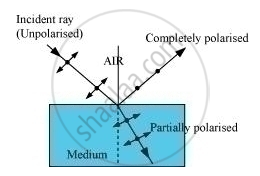PUC Karnataka Science Class 12Department of Pre-University Education, Karnataka
Share

# What is an Unpolarized Light? Explain with the Help of Suitable Ray Diagram How an Unpolarized Light Can Be Polarized by Reflection from a Transparent Medium. - PUC Karnataka Science Class 12 - Physics

#### Question

What is an unpolarized light? Explain with the help of suitable ray diagram how an unpolarized light can be polarized by reflection from a transparent medium. Write the expression for Brewster angle in terms of the refractive index of denser medium.

#### SolutionAn unpolarised light is one in which the vibration of electric field vector is not restricted in one particular plane.

When an unpolarised light falls on the surface, the reflected light is such that the vibration of its electric field vector is confined to one particular plane. The direction of this plane is parallel to the surface of reflection.

A component of electric field vector is absent from the refracted light. Therefore, the refracted light is partially polarised.

The expression for Brewster angle in terms of the refractive index of denser medium is tani_B = mu

Where, μ is the refractive index of the denser medium with respect to the rarer medium.

Is there an error in this question or solution?

#### Video TutorialsVIEW ALL 

Solution What is an Unpolarized Light? Explain with the Help of Suitable Ray Diagram How an Unpolarized Light Can Be Polarized by Reflection from a Transparent Medium. Concept: Plane Polarised Light.
S